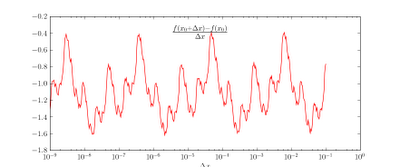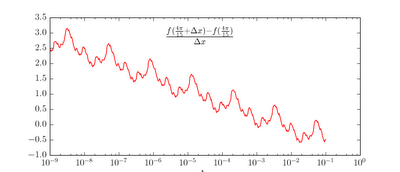### The 36π/127 conjecture

Update: the conjecture is apparently wrong.
There is a problem that bothers me for a long time. Consider function f(x), defined in the following way:
$$f(x) = \sum_{n=0}^\infty \frac{sin(2^n x)}{2^n}.$$
Obviously, this sum converges for every real x; and for every x, -2 < f(x) < 2; and f(x) is continuous (due to the uniform convergence of partial sums).
The special thing about f(x) is that it has no derivatives. It may be easily seen from it's graph:
The red line in the graph is f(x), is obviously a fractal. Using box-counting approach, it's dimension may be approximated as 1.03, though this value tends to decrease, when minimal box size is decreased.
Using numeric methods, I have found that f(x) achieves it's maximal value at point xmax = 0.8905302... (dash line at the graph above) which effectively equals to $$x_0=\frac{36}{127}\pi$$. I am looking for the proof of this fact, but without success.
Following plot may give a key to the solution. It is a logarithmic graph, showing how the function behaves in the close vicinity of the point $$x_0$$.Graph of the f(x) in the vicinity of the point x0.
The notable feature of this graph is its periodicity. At other points, f(x) have more complex behavior:Periodical structure appears only if x is a rational fraction of $$\pi$$. For other values, graph shows chaotic structure: